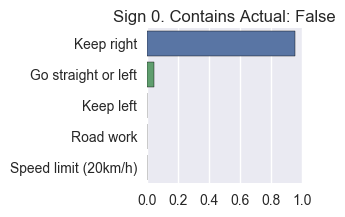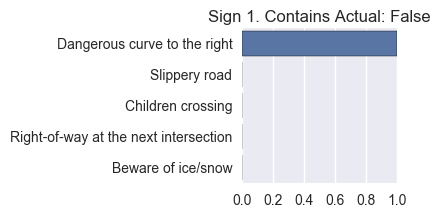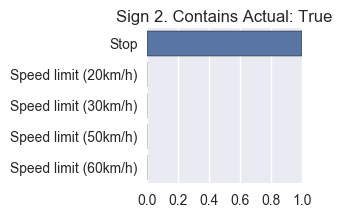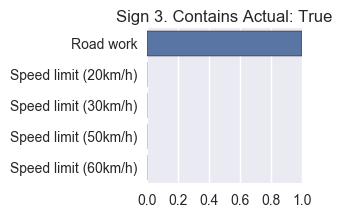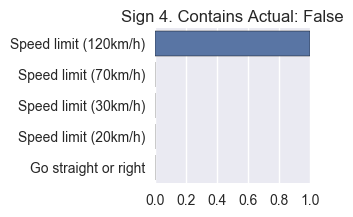# Self-Driving Car Engineer Nanodegree¶

## Project: Build a Traffic Sign Recognition Classifier¶

In this notebook, a template is provided for you to implement your functionality in stages which is required to successfully complete this project. If additional code is required that cannot be included in the notebook, be sure that the Python code is successfully imported and included in your submission, if necessary. Sections that begin with 'Implementation' in the header indicate where you should begin your implementation for your project. Note that some sections of implementation are optional, and will be marked with 'Optional' in the header.

Note: Code and Markdown cells can be executed using the Shift + Enter keyboard shortcut. In addition, Markdown cells can be edited by typically double-clicking the cell to enter edit mode.

## Step 0: Load The Data¶

In :
# Load pickled data
import pickle
import tensorflow as tf

# TODO: Fill this in based on where you saved the training and testing data

training_file = "./data/train.p"
testing_file = "./data/test.p"

with open(training_file, mode='rb') as f:
with open(testing_file, mode='rb') as f:

X, y = train['features'], train['labels']
X_test, y_test = test['features'], test['labels']


## Step 1: Dataset Summary & Exploration¶

The pickled data is a dictionary with 4 key/value pairs:

• 'features' is a 4D array containing raw pixel data of the traffic sign images, (num examples, width, height, channels).
• 'labels' is a 2D array containing the label/class id of the traffic sign. The file signnames.csv contains id -> name mappings for each id.
• 'sizes' is a list containing tuples, (width, height) representing the the original width and height the image.
• 'coords' is a list containing tuples, (x1, y1, x2, y2) representing coordinates of a bounding box around the sign in the image. THESE COORDINATES ASSUME THE ORIGINAL IMAGE. THE PICKLED DATA CONTAINS RESIZED VERSIONS (32 by 32) OF THESE IMAGES

Complete the basic data summary below.

In :
### Replace each question mark with the appropriate value.
import numpy as np

# TODO: Number of training examples
n_train = X.shape

# TODO: Number of testing examples.
n_test = X.shape

# TODO: What's the shape of an traffic sign image?
image_shape = X.shape[1:]

# TODO: How many unique classes/labels there are in the dataset.
n_classes = np.unique(y).shape

print("Number of training examples =", n_train)
print("Number of testing examples =", n_test)
print("Image data shape =", image_shape)
print("Number of classes =", n_classes)

Number of training examples = 39209
Number of testing examples = 39209
Image data shape = (32, 32, 3)
Number of classes = 43


Visualize the German Traffic Signs Dataset using the pickled file(s). This is open ended, suggestions include: plotting traffic sign images, plotting the count of each sign, etc.

The Matplotlib examples and gallery pages are a great resource for doing visualizations in Python.

NOTE: It's recommended you start with something simple first. If you wish to do more, come back to it after you've completed the rest of the sections.

In :
### Data exploration visualization goes here.
### Feel free to use as many code cells as needed.
import matplotlib.pyplot as plt
import seaborn as sns
# Visualizations will be shown in the notebook.
%matplotlib inline

In :
plt.figure(figsize=(15,5))
sns.countplot(y)
plt.title("Distribution of Training Labels")

Out:
<matplotlib.text.Text at 0x12a6a2400>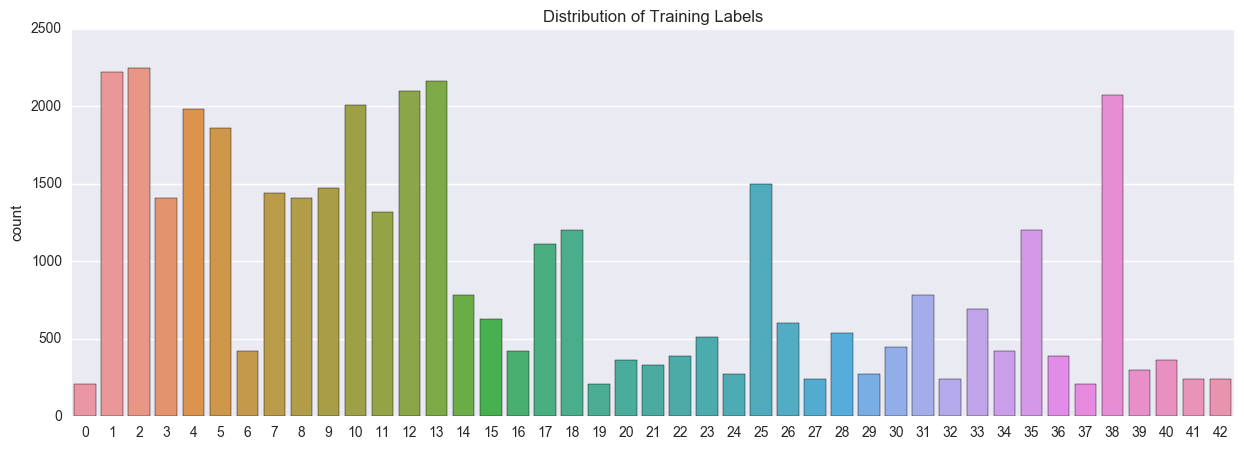In :
plt.imshow(X)

Out:
<matplotlib.image.AxesImage at 0x12e067128>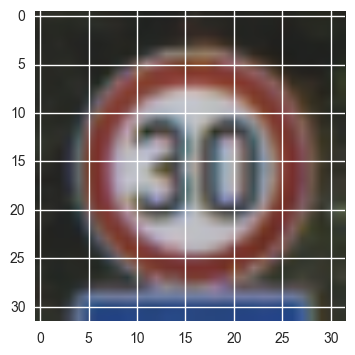## Step 2: Design and Test a Model Architecture¶

Design and implement a deep learning model that learns to recognize traffic signs. Train and test your model on the German Traffic Sign Dataset.

• Neural network architecture
• Play around preprocessing techniques (normalization, rgb to grayscale, etc)
• Number of examples per label (some have more than others).
• Generate fake data.

Here is an example of a published baseline model on this problem. It's not required to be familiar with the approach used in the paper but, it's good practice to try to read papers like these.

NOTE: The LeNet-5 implementation shown in the classroom at the end of the CNN lesson is a solid starting point. You'll have to change the number of classes and possibly the preprocessing, but aside from that it's plug and play!

### Implementation¶

Use the code cell (or multiple code cells, if necessary) to implement the first step of your project. Once you have completed your implementation and are satisfied with the results, be sure to thoroughly answer the questions that follow.

In :
### Preprocess the data here.
### Feel free to use as many code cells as needed.
import cv2

def convert_to_yuv(images):
images_y = np.array([cv2.cvtColor(img, cv2.COLOR_BGR2YUV) for img in images])
return images_y

def norm_images(images):
return np.multiply(images, 1.0 / 255.0)

def preprocess_images(images):
return norm_images(convert_to_yuv(images))

X_pre = preprocess_images(X)
X_test_pre = preprocess_images(X_test)


### Question 1¶

Describe how you preprocessed the data. Why did you choose that technique?

I preprocessed the data by converting the images to YUV. I chose to do this after reading the cited paper below and seeing that they chose to convert to YUV. I did some additional research and it turns on that this conversion might not be completely necessary, but found no evidence that it would make the process worse. Also, there is some research that suggests YUV is less prone to adversarial perturbations.

Also, I then normalized the pixel data to be between 1 and 0 by dividing all the values by 255. I choose these techniques based on the "Traffic Sign Recognition with Multi-Scale Convolutional Networks" as these seemed to work well as preprocessing steps. Also, I new it would be important to normalize the data to help gradient descent perform better.

In :
### Generate data additional data (OPTIONAL!)
### and split the data into training/validation/testing sets here.
### Feel free to use as many code cells as needed.

def rescale(img):
scale_factor = np.random.uniform(.9, 1.1)
if scale_factor > 1:
inter_type = cv2.INTER_LINEAR
else:
inter_type = cv2.INTER_AREA
return cv2.resize(img,None,fx=scale_factor, fy=scale_factor, interpolation = inter_type)

def rotate(img):
rows,cols,_ = img.shape
rotate_factor = np.random.uniform(-15.0, 15.0)
M = cv2.getRotationMatrix2D((cols/2,rows/2),rotate_factor,1)
return cv2.warpAffine(img,M,(cols,rows))

def translate(img):
rows,cols,_ = img.shape
x_translate_factor = np.random.uniform(-2.0, 2.0)
y_translate_factor = np.random.uniform(-2.0, 2.0)
M = np.float32([[1,0,x_translate_factor],[0,1,y_translate_factor]])
return cv2.warpAffine(img,M,(cols,rows))

def jitter_data(data, labels, scale=5):
assert data.shape == labels.shape
shape = data.shape
jitter_data = np.zeros((shape*scale,shape,shape,shape))
jitter_labels = np.zeros(shape*scale)
for n in range(scale):
start_index = n * shape
for i in range(shape):
img = rescale(data[i,:,:,:])
img = rotate(img)
img = translate(img)
row, col, _ = img.shape
if row > 32:
inter_type = cv2.INTER_LINEAR
else:
inter_type = cv2.INTER_AREA
img = cv2.resize(img,(32, 32), interpolation = inter_type)
jitter_data[start_index+i,:,:,:] = img
jitter_labels[start_index+i] = labels[i]
return jitter_data, jitter_labels

In :
X_train_jitter, y_train_jitter = jitter_data(X_pre, y, scale=3)
X_train_all = np.concatenate((X_train_jitter, X_pre), axis=0)
y_train_all = np.append(y_train_jitter, y)

In :
from sklearn.model_selection import train_test_split
from sklearn.utils import shuffle

X_train, X_validation, y_train, y_validation = train_test_split(X_train_all, y_train_all, test_size=0.20, random_state=42)
X_train, y_train = shuffle(X_train, y_train)


### Question 2¶

Describe how you set up the training, validation and testing data for your model. Optional: If you generated additional data, how did you generate the data? Why did you generate the data? What are the differences in the new dataset (with generated data) from the original dataset?

I left the testing set as was given to us and split the training data into training and validation. To do this I used scikit learn's train test split functionality using 20% for the validation set and 80% for the training set.

I generated data in the same way as, "Traffic Sign Recognition with Multi-Scale Convolutional Networks." I created 3 extra versions of each image by slightly perturbing the scale, rotation, and translation of the image. I generated the data because adding some distortion to our training data will help our model be more robust.

In :
### Define your architecture here.
### Feel free to use as many code cells as needed.
from tensorflow.contrib.layers import flatten

def conv_layer(x, shape, strides, padding, mean, stddev):
W = tf.Variable(tf.truncated_normal(shape, mean=mean, stddev=stddev))
b = tf.Variable(tf.zeros(shape[-1]))
return tf.nn.relu(conv)

def fully_connected(x, shape, mu, sigma, actiavation=True):
W = tf.Variable(tf.truncated_normal(shape, mean=mu, stddev=sigma))
b = tf.Variable(tf.zeros(shape[-1]))
fc = tf.matmul(x, W) + b
if actiavation:
return tf.nn.relu(fc)
else:
return fc

def LeNet(x):
# Hyperparameters
mu = 0
sigma = 0.1

conv1 = conv_layer(x, (5,5,3,6), [1,1,1,1], padding, mu, sigma)
conv1 = tf.nn.max_pool(conv1, ksize=[1, 2, 2, 1], strides=[1, 2, 2, 1], padding=padding)

conv2 = conv_layer(conv1, (5,5,6,16), [1,1,1,1], padding, mu, sigma)
conv2 = tf.nn.max_pool(conv2, ksize=[1, 2, 2, 1], strides=[1, 2, 2, 1], padding='VALID')

fc0   = flatten(conv2)
fc1   = fully_connected(fc0, (400, 120), mu, sigma)
fc2   = fully_connected(fc1, (120, 84), mu, sigma)
return fully_connected(fc2, (84, 43), mu, sigma, False)

def alex_net(x):
# Hyperparameters
mu = 0
sigma = 0.1

layer_1 = conv_layer(x, (4,4,3,14), [1,1,1,1], padding, mu, sigma)
#layer_1 = tf.nn.local_response_normalization(layer_1)

layer_2 = conv_layer(layer_1, (2,2,14,38), [1,1,1,1], padding, mu, sigma)
#layer_2 = tf.nn.local_response_normalization(layer_2)

#layer_3 = conv_layer(layer_2, (2,2,38,57), [1,1,1,1], padding, mu, sigma)
#layer_4 = conv_layer(layer_3, (2,2,57,57), [1,1,1,1], padding, mu, sigma)

layer_5 = conv_layer(layer_2, (2,2,38,38), [1,1,1,1], padding, mu, sigma)
#layer_5 = tf.nn.local_response_normalization(layer_5)

fc0 = flatten(layer_5)
network_shape = (fc0.get_shape().as_list()[-1], 4096)

fc1 = fully_connected(fc0, network_shape, mu, sigma)
fc1 = tf.nn.dropout(fc1, dropout_keep_prob)

fc2 = fully_connected(fc1, (4096,4096), mu, sigma)
fc2 = tf.nn.dropout(fc2, dropout_keep_prob)

return fully_connected(fc2, (4096, 43), mu, sigma, False)


### Question 3¶

What does your final architecture look like? (Type of model, layers, sizes, connectivity, etc.) For reference on how to build a deep neural network using TensorFlow, see Deep Neural Network in TensorFlow from the classroom.

I modeled my final architecture off of AlexNet. It is not exactly the same, but I used most of the architecture in a scaled down manner.

All weights are initialized using a truncated normal with a mean of 0 and a standard deviation of 0.1. All biases are initialized with zeros. The input dimension is 32X32X3.

I start with a 14 layer deep convolutional layer with relu activation and then max pooling. I use a filter size of 4x4 with a stride of 1 and valid padding for the convolutional layer. For max pooling, I use a 2x2 filter with a stride of 2 in both directions.

I then repeat this process again, but with a depth of 38 on the convolutional layer and a filter size of 2x2.

I then repeat exactly the layer above with the depth of 38.

I then flatten to a fully connected layer of size 4096 and relu activation followed by drop out with a 50% probability. I repeat this layer again and then output the logits.

In :
x_tf = tf.placeholder(tf.float32, (None, 32, 32, 3))
y_tf = tf.placeholder(tf.int32, (None))
dropout_keep_prob = tf.placeholder(tf.float32)
one_hot_y = tf.one_hot(y_tf, 43)

rate = 0.001

logits = alex_net(x_tf)
cross_entropy = tf.nn.softmax_cross_entropy_with_logits(logits, one_hot_y)
loss_operation = tf.reduce_mean(cross_entropy)
training_operation = optimizer.minimize(loss_operation)

correct_prediction = tf.equal(tf.argmax(logits, 1), tf.argmax(one_hot_y, 1))
accuracy_operation = tf.reduce_mean(tf.cast(correct_prediction, tf.float32))
saver = tf.train.Saver()

In :
def evaluate(X_data, y_data, BATCH_SIZE):
num_examples = len(X_data)
total_accuracy = 0
sess = tf.get_default_session()
for offset in range(0, num_examples, BATCH_SIZE):
batch_x, batch_y = X_data[offset:offset+BATCH_SIZE], y_data[offset:offset+BATCH_SIZE]
accuracy = sess.run(accuracy_operation, feed_dict={x_tf: batch_x, y_tf: batch_y, dropout_keep_prob:1.0})
total_accuracy += (accuracy * len(batch_x))

In :
EPOCHS = 50
BATCH_SIZE = 128
DROP_PROB = 0.5
SAVE_FILE = 'alex_net'
train = False

if train:
with tf.Session() as sess:
sess.run(tf.global_variables_initializer())
num_examples = len(X_train)

print("Training...")
print()
for i in range(EPOCHS):
X_train, y_train = shuffle(X_train, y_train)
for offset in range(0, num_examples, BATCH_SIZE):
end = offset + BATCH_SIZE
batch_x, batch_y = X_train[offset:end], y_train[offset:end]
sess.run(training_operation, feed_dict={x_tf: batch_x, y_tf: batch_y, dropout_keep_prob: DROP_PROB})

validation_accuracy = evaluate(X_validation, y_validation, BATCH_SIZE)
print("EPOCH {} ...".format(i+1))
print("Validation Accuracy = {:.3f}".format(validation_accuracy))
print()

saver.save(sess, SAVE_FILE)
print("Model saved")


### Question 4¶

How did you train your model? (Type of optimizer, batch size, epochs, hyperparameters, etc.)

I used the Adam optimizer with a learning rate of 0.001. I used a batch size of 128 and 50 epochs. I also initialized my weights using a truncated normal with mu = 0 and sigma = 0.1. Lastly, I used padding = 'VALID.'

I decided on the number of ephochs via trial and error. I first started with 100 epochs and then realized I was overfitting to the data. So I then scaled down the number and watched it by priniting the validation accuracy after each epoch to see when the validation accuracy started to level off. At that point (about 50), I stop the training and saved the weights. For future projects, I may write code to automatically stop the training for me, so I don't have to watch long training networks.

### Question 5¶

What approach did you take in coming up with a solution to this problem? It may have been a process of trial and error, in which case, outline the steps you took to get to the final solution and why you chose those steps. Perhaps your solution involved an already well known implementation or architecture. In this case, discuss why you think this is suitable for the current problem.

My approach was fairly straight forward. I stared with the implementation of LeNet from a previous lab to make sure I could get the model working as expected. Once I had LeNet working on the traffic sign data, I took a look at some of the other popular convolutional architectures. After AlexNet and ZFNet, the architectures seemed to become too deep and complicated to implement easily in TensorFlow, so I settled on AlexNet. In order to train the network faster, I scaled down the depth of the convolutional layers of AlexNet. My first attempt, though, seemed to drastically overfit to the training/validation data as it performed very well on validation data but not testing. To account for this I removed some of the convolutional layers and the local response normalization layers.

I think an AlexNet like architecture was suitable for the current problem because AlexNet performed very well on ImageNet and was still manageable for me to implement. AlexNet is also a very popular architecture that has been used many times with good results, so it was a great starting place for me and allowed me to leverage the research that went into its creation.

## Step 3: Test a Model on New Images¶

Take several pictures of traffic signs that you find on the web or around you (at least five), and run them through your classifier on your computer to produce example results. The classifier might not recognize some local signs but it could prove interesting nonetheless.

You may find signnames.csv useful as it contains mappings from the class id (integer) to the actual sign name.

### Implementation¶

Use the code cell (or multiple code cells, if necessary) to implement the first step of your project. Once you have completed your implementation and are satisfied with the results, be sure to thoroughly answer the questions that follow.

In :
with tf.Session() as sess:
new_saver = tf.train.import_meta_graph('alex_net.meta')
new_saver.restore(sess, tf.train.latest_checkpoint('./'))
test_accuracy = evaluate(X_test_pre, y_test, 128)
print("Test accuracy: {}".format(test_accuracy))

Test accuracy: 0.9395091051538125

In :
### Load the images and plot them here.
### Feel free to use as many code cells as needed.

img = cv2.cvtColor(img, cv2.COLOR_BGR2RGB)
return cv2.resize(img,(32, 32), interpolation = cv2.INTER_AREA )


In :
sign_batch = preprocess_images(np.stack([sign_0, sign_1, sign_2, sign_3, sign_4], 0))
sign_truth = [34, 31, 14, 25, 21]

In :
with tf.Session() as sess:
new_saver = tf.train.import_meta_graph('alex_net.meta')
new_saver.restore(sess, tf.train.latest_checkpoint('./'))
sign_accuracy = evaluate(sign_batch, sign_truth, 5)
sign_predictions = sess.run(tf.argmax(logits, 1), feed_dict={x_tf: sign_batch, y_tf: sign_truth, dropout_keep_prob: 1})
top_5 = sess.run(tf.nn.top_k(tf.nn.softmax(logits), 5), feed_dict={x_tf: sign_batch, y_tf: sign_truth, dropout_keep_prob: 1})
print("Sign accuracy: {}".format(sign_accuracy))

Sign accuracy: 0.4000000059604645


### Question 6¶

Choose five candidate images of traffic signs and provide them in the report. Are there any particular qualities of the image(s) that might make classification difficult? It could be helpful to plot the images in the notebook.

In :
plt.imshow(sign_0)

Out:
<matplotlib.image.AxesImage at 0x114d19e80>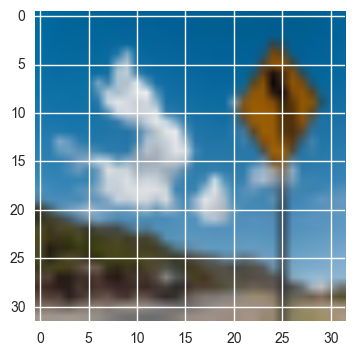In :
plt.imshow(sign_1)

Out:
<matplotlib.image.AxesImage at 0x114d9b4a8>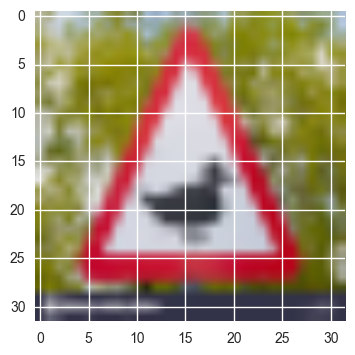In :
plt.imshow(sign_2)

Out:
<matplotlib.image.AxesImage at 0x11634a6a0>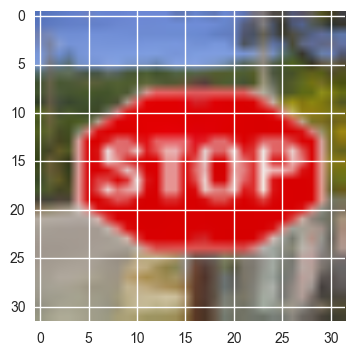In :
plt.imshow(sign_3)

Out:
<matplotlib.image.AxesImage at 0x1163caac8>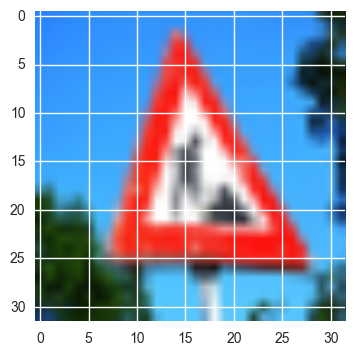In :
plt.imshow(sign_4)

Out:
<matplotlib.image.AxesImage at 0x116451198>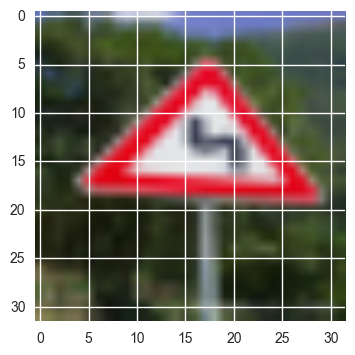There are a few things with some of the images that might make them hard to classify. Sign 0 has some background in the photo as opposed to just a straight photo of the sign. Also, sign 1 is potentially not in our dataset. We do have a category for wild animal crossing, but that category is a picture of a deer and not a duck (as seen below). The other images seem fairly straight forward except for the fact that down sampling them to 32x32 distored the image a bit.

In :
### Run the predictions here.
### Feel free to use as many code cells as needed.

wild_indexes = [i for i in range(len(y)) if y[i] == 31]
plt.imshow(X[wild_indexes])

Out:
<matplotlib.image.AxesImage at 0x12d531710>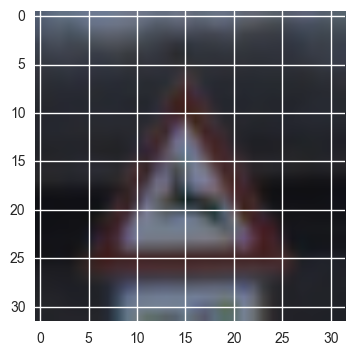### Question 7¶

Is your model able to perform equally well on captured pictures when compared to testing on the dataset? The simplest way to do this check the accuracy of the predictions. For example, if the model predicted 1 out of 5 signs correctly, it's 20% accurate.

NOTE: You could check the accuracy manually by using signnames.csv (same directory). This file has a mapping from the class id (0-42) to the corresponding sign name. So, you could take the class id the model outputs, lookup the name in signnames.csv and see if it matches the sign from the image.

From my code above, one can see that I achieved a 40% accuracy on my captured pictures as opposed to a 93.95% accuracy on the test set. I correctly classified sign 2 and 3. These seemed to be the most simple captured images to classify, so that doesn't surprise me.

### Question 8¶

Use the model's softmax probabilities to visualize the certainty of its predictions, tf.nn.top_k could prove helpful here. Which predictions is the model certain of? Uncertain? If the model was incorrect in its initial prediction, does the correct prediction appear in the top k? (k should be 5 at most)

tf.nn.top_k will return the values and indices (class ids) of the top k predictions. So if k=3, for each sign, it'll return the 3 largest probabilities (out of a possible 43) and the correspoding class ids.

Take this numpy array as an example:

# (5, 6) array
a = np.array([[ 0.24879643,  0.07032244,  0.12641572,  0.34763842,  0.07893497,
0.12789202],
[ 0.28086119,  0.27569815,  0.08594638,  0.0178669 ,  0.18063401,
0.15899337],
[ 0.26076848,  0.23664738,  0.08020603,  0.07001922,  0.1134371 ,
0.23892179],
[ 0.11943333,  0.29198961,  0.02605103,  0.26234032,  0.1351348 ,
0.16505091],
[ 0.09561176,  0.34396535,  0.0643941 ,  0.16240774,  0.24206137,
0.09155967]])

Running it through sess.run(tf.nn.top_k(tf.constant(a), k=3)) produces:

TopKV2(values=array([[ 0.34763842,  0.24879643,  0.12789202],
[ 0.28086119,  0.27569815,  0.18063401],
[ 0.26076848,  0.23892179,  0.23664738],
[ 0.29198961,  0.26234032,  0.16505091],
[ 0.34396535,  0.24206137,  0.16240774]]), indices=array([[3, 0, 5],
[0, 1, 4],
[0, 5, 1],
[1, 3, 5],
[1, 4, 3]], dtype=int32))

Looking just at the first row we get [ 0.34763842, 0.24879643, 0.12789202], you can confirm these are the 3 largest probabilities in a. You'll also notice [3, 0, 5] are the corresponding indices.

As can be seen below, my network is very confident in all of its predictions. Which is somewhat unfortunate because it only gets 40% correct. The model was either correct in its prediction or the correct prediction did not appear in the top 5 predictions.

Note: Once you have completed all of the code implementations and successfully answered each question above, you may finalize your work by exporting the iPython Notebook as an HTML document. You can do this by using the menu above and navigating to \n", "File -> Download as -> HTML (.html). Include the finished document along with this notebook as your submission.

In :
import pandas as pd


In :
def get_class_names(probabilities, classes, class_map):
class_names = []
for i in range(len(probabilities)):
key = class_map[class_map.ClassId == classes[i]].SignName.values
class_names.append(key)
return class_names

def get_plots(top_5, class_map, actuals):
n = len(top_5)
for i in range(n):
truth = actuals[i]
is_in_top_5 = truth in top_5[i]
class_name = get_class_names(top_5[i], top_5[i], class_map)
probabilities = top_5[i]
plt.figure(figsize=(2,2))
plt.title("Sign {0}. Contains Actual: {1}".format(i, is_in_top_5))
sns.barplot(probabilities, class_name)

In :
get_plots(top_5, class_map, sign_truth)